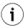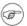On-line GuidesAll GuideseBook StoreiOS / AndroidLinux for BeginnersOffice ProductivityLinux InstallationLinux SecurityLinux UtilitiesLinux VirtualizationLinux KernelSystem/Network AdminProgrammingScripting LanguagesDevelopment ToolsWeb DevelopmentGUI Toolkits/DesktopDatabasesMail SystemsopenSolarisEclipse DocumentationTechotopia.comVirtuatopia.comHow To GuidesVirtualizationGeneral System AdminLinux SecurityLinux FilesystemsWeb ServersGraphics & DesktopPC HardwareWindowsProblem SolutionsPrivacy Policy# Chapter 12. Mathematical tools

 num-utils homepageThe "num-utils" homepage, Num Utils, contains a variety of command line programs that could be useful when performing maths on your GNU/Linux machine.

units Convert units of measurement between different scales. For example, centimeters to inches, litres to gallons.

Simply run the program, I recommend running it as follows:

 ```units --verbose ```

This will run the program and it will tell you exactly what it is doing.

Example: you enter "60 meters" then you want it worked out in "kilometers". The first line will tell you what this evaluates to.

If you wanted the conversion rate for "meters" to "kilometers" read the second line of the output (which will tell you meters/1000).

Note: press CTRL-D (end-of-file key) when you are finished using units.

python Python is a very powerful, easy to learn, general purpose, interpreted programming language. .h and it makes a great calculator! If you don't have a calculator installed then simply type python, then hit [Enter].

This will execute the Python interpreter in interactive mode. Type your sums just like you would use a calculator. Note that if you want to work out fractions make sure you use a decimal point and a zero to obtain the correct answer (otherwise it will use integer division).

To start python in interactive mode, simply type:

 ```python ```

Once python is started you can use it to add up sums or maybe do some python programming.

Use CTRL-D (end-of-file key) to exit the Python interpreter.

numgrep A little bit like grep only this is designed for numbers only.

Use '/' (forward slashes) to contain each expression.

Use m<n> to find multiples of the number n and use f<n> to find factors of the number n.

Use commas to seperate expressions and .. (two dots) to represent a range.

For example, to input from standard input you could simply type:

 ```numgrep ```

To input from a file and look for numbers between 1 and 1000 you could type:

 ```numgrep /1..1000/ file_name ```
 This tool comes from the num-utils packagePlease note that this tool is part of the num-utils package.Published under the terms of the GNU General Public License Design by Interspire